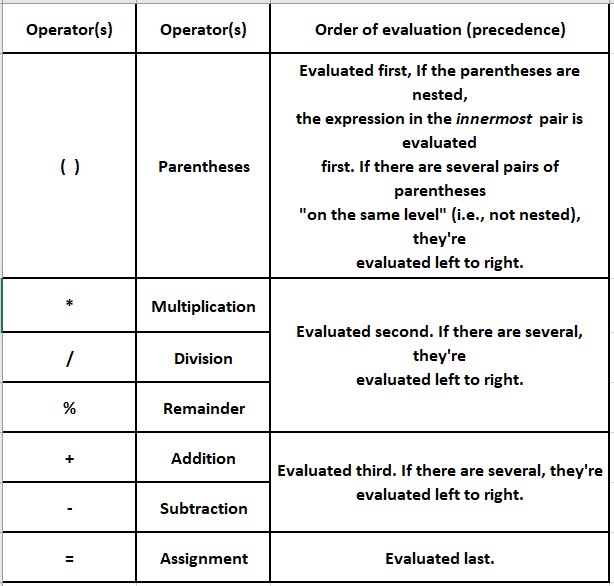In this tutorial, we are going to learn about the operator precedence, and associativity.

## Operator Precedence & Associativity:

When several operators appear in one expression, evaluation takes place according to certain predefined hierarchical rules.

These rules specify the order of evaluation (also called precedence) which determines the priority of operators.

The operator with higher precedence is evaluated before others with lesser precedence.

Consider the following expression: a = 2 + 3 * 4 – 4 / 2 + 6. It is evaluated depending on the precedence rules of the operators.

``````a = 2 + 3 * 4 - 4 / 2 + 6 //3 * 4 is evaluaed first because * and / have the highest precedence
a = 2 + 12 - 4 / 2 + 6 //4 / 2 is evaluated next
a = 2 + 12 - 2 + 6 //+, - are evaluaed simulatneously since they have same precedence``````

Associativity or grouping refers to the order in which C evaluates the operations that have the same precedence level. All operators can associate either from right to left or from left to right.

Parentheses may be included in an expression to change the order of evaluation. It forces the enclosed operations to be performed first because parentheses are given the highest precedence.

For example, consider the following expression:

``a = (2 + 3) - (4 - 5) * 6 * (6 % 2)``

The expressions included in parentheses are evaluated first and then, the remaining operators are evaluated.

``````a = (5) - (-1) * 6 * 0

a = (5) - (-1) * 0

a = 5 - 0 = 5``````

Parentheses are used in C expressions in the same manner as in algebraic expressions. For example, to multiply times the quantity b+c we write a *(b+c).

## Rules of Operator Precedence:

C applies the operators in arithmetic expressions in a precise sequence determined by the following rules of operator precedence, which are generally the same as those in algebra:

1. Operators in expressions contained within pairs of parentheses are evaluated first. First Parentheses are said to be at the “highest level of precedence”. In the case of nested, or embedded parentheses, such as ((a + b)+ c).
2. Multiplication, division, and remainder operations are applied next. If an expression contains several multiplications, division, and remainder operations, evaluation proceeds from left to right. Multiplication, division, and the remainder are said to be on the same level of precedence.
3. Addition and Subtraction operations are evaluated next. If an expression contains several additions and subtraction operations, evaluation proceeds from left to right. Addition and Subtraction also have the same level of precedence, which is lower than the precedence of the multiplication, division, and remainder operations.
4. The Assignment operator (=) is evaluated first.

The rules of operator precedence specify the order C uses to evaluate expressions. When we say evaluation proceeds from left to right, we’re referring to the associativity of the operators.

For reference, use the following table.## References:

Happy Learning### About the Author: Hemanth Krishna Vineel RamayanamI’m an Undergraduate Student at the Indian Institute of Information Technology, Design, and Manufacturing, Kurnool, Andhra Pradesh. I belong to the CSE domain.